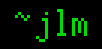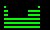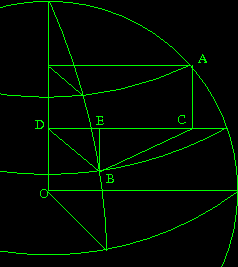## 31-Jul-2008

### A-A-B-B-A

Filed under: humor — jlm @ 12:40

I’ll give you up only when the sun’s cold
And my hurting you is forbode
Shan’t ever run aroun’
Nor ever let you down
You’ve just been limerickrolled.

## 24-Jun-2008

### 2000 in 1910

Filed under: misc — jlm @ 12:07

It’s the twenty-first century. Where are the flying cars? The videophones? What about the robot barbers and tailor engines? I’m kind of glad we didn’t get radium fireplaces though.

## 3-Apr-2008

### Regex breakpoints in gdb

Filed under: programming — jlm @ 12:49

gdb has this awesome feature that nobody seems to have heard of (including myself before today). You can set breakpoints by regex!

“help rbreak”

## 14-Mar-2008

### Archimedes simplified

Filed under: math — jlm @ 12:15

In the third century BC, Archimedes calculated an amazing approximation to π, bettering the old value of 256/81 worked out by 17th century Egyptians.
How did he do it? He circumscribed and inscribed a hexagon around a circle, then bisected each hexagon’s central angles to make a dodecagon, then a 24-gon, 48-gon, and finally a 96-gon. The perimeters of the polygons bound π above and below, and each pair of perimeters is related to the ones before.
Consider a pair of polygons being bisected:If the radius AO = 1, then AB = tan α and AC = tan ½α.
If it has n sides, the outer perimeter P = 2n tan α and the new polygon has perimeter P’ = 4n tan ½α. The inner perimeter is p = 2n sin α, with the new polygon’s perimeter being p’ = 4n sin ½α.

Now, 1/P + 1/p = 1/(2n tan α) + 1/(2n sin α) = (sin α + tan α)/(2n sin α tan α) = (1 + sec α)/(2n tan α) = (cos α + 1)/(2n sin α) = (cos² ½α – sin² ½α + 1)/(4n sin ½α cos ½α) = (2 cos² ½α – 1 + 1)/(4n sin ½α cos ½α) = (1/2n) cot ½α = 2/P’
And, P’ p = (4n tan ½α)(2n sin α) = 16n² tan ½α sin ½α cos ½α = 16n² sin² ½α = p’²
So, we have easy ways to calculate successive perimeters from our basic hexagons, with p = 6, P = 4 √3, using only simple arithmetic and square roots.

n P p
6 6.928203 6
12 6.430781 6.211657
24 6.319320 6.265257
48 6.292172 6.278700
96 6.285429 6.282064

which puts 3.1427 > π > 3.1410.

Now, Archimedes didn’t have trigonometric functions to utilize in his calculations, so he calculated the perimeters using similar triangles, a much more complicated process. But it gives the same values. He also lacked decimals, so he worked with rationals instead, producing the bounds 3 1/7 > π > 3 10/71.

## 4-Mar-2008

### Song chart

Filed under: humor, math — jlm @ 20:18

I recently was amused by the “song chart” meme, so decided to give it a try.## 28-Jan-2008

### Newcomb’s Paradox, part II

Filed under: philosophy — jlm @ 10:46

It’s been pointed out I’m not really addressing what’s wrong with the two arguments which give you different “correct” choices for the Newcomb scenario. So here we go…

Expected Outcome
This argument assumes that the predictor has foreseen our choice [with high probability]. If however we assume that free willed choices are by definition unpredictable, this is a logical impossibility.

Dominant Outcome
This argument assumes that the choice we make is not correlated with the predictor’s choice, because that would involve reverse causation. However, if we assume that we’re not free willed, or that freely willed choices can be predicted (whatever that would involve), then there’s a common cause: Our mind’s tendency to select choice X in the scenario causes both our selection of X when we’re in the scenario and the predictor to anticipate this selection. So, our choice wouldn’t be independent of the predictor’s, and dominance wouldn’t hold.

## 27-Jan-2008

### Musings on Newcomb’s Paradox

Filed under: philosophy — jlm @ 11:45

So, I’ve been thinking about Newcomb’s Paradox lately.
I think part of the issue is it conflates a couple issues, and it might be useful to consider them separately. So, think on these paradoxes:

Determinism vs. Nondeterminism
Consider a superhuman predictor and a fair coin. The predictor predicts what the coin will show, then you flip it. The predictor is [nearly] always right.

Removal of free will
A computer has been programmed to maximize its expected score as a player in the Newcomb scenario, given that the predictor has a copy of the program to analyze, run in simulation or on another computer, etc. How will it play?

My take on Newcomb’s paradox? It reduces to the question of whether free will makes our choices inherently unpredictable, and the paradox is thorny because free will isn’t well defined enough to provide a clear answer.
If we have no free will, it’s just the computer scenario. If we assume free willed actions are inherently unpredictable, then the existence of a predictor contradicts that assumption, just like it contradicts the assumption that the outcome of a fair coin flip cannot be predicted.

## 10-May-2007

### Tip: compression

Filed under: general — jlm @ 16:28

It doesn’t help to zip already compressed file formats, like jpeg or mpeg, it only wastes CPU. Uncompressed video, audio, or still images will compress better with algorithms which are dedicated for video, audio, or images than they will with general compressors. JPEG is for photos — for images which are line drawings use PNG.

## 3-May-2007

### Periodic table of *

Filed under: science — jlm @ 13:36Okay, so the Periodic Table of the Elements is one of the iconic symbols of Chemistry. Even if you flunked your high school Chem, you’ll recognize it instantly. Unfortunately, if you flunked high school Chem, you won’t actually know what it means.

Not recognizing that it’s a systematic arrangement of all the chemical elements, elucidating their properties in terms of their electron structure, you’re tempted to fill it in instead with items from whatever interest you do have. Make a Periodic Table of Fruit, or a Periodic Table of Government, or a Periodic Table of Rhetoric, then feel like you’ve somehow contributed something useful to Fruit classification. But what does this non-Elemental table mean? Do the properties of fruit recur periodically as something corresponding to atomic number increases? What even corresponds to atomic number? Why should fruit group as if it had electron shells? Does your table actually explain anything? Or are you just capitalizing on the fact that atoms do have electron shells and so elemental properties do arrange into a periodic table which is tremendously useful for chemistry, and applying it to something completely inappropriate?

## 30-Mar-2007

### Distance between points on a sphere

Filed under: math — jlm @ 15:22

If you try and find out how you compute the distance between points on a sphere, you’ll get a bunch of sites which offer to calculate it for you if you enter the coordinates. If you search harder, you can even get the formula. But no one seems to be offering a derivation. So here you go.

First, some preliminaries. The half angle formulas give us 2 sin² ½ψ = 1-cos ψ. (Easily derivable from cos 2α = cos² α – sin² α.) The straight-line distance (cutting through the inside of the sphere) between two points on a unit sphere with an angle ψ between them is 2 sin ½ψ. (Bisect the triangle formed by the two points and the center of the sphere.)Consider A and B to be our two points we want to get the distance between, with latitudes φA and φB, and longitudes which differ by Θ. We’ll operate mostly on the disk formed by the parallel through B. The point where it intersects the pole is D, and the projection of A onto it is C. Let EB be perpendicular to DC.

The radius of the parallel through A is cos φA = DC; the one through B is cos φB = DB. Angle CDB is Θ, so EB = rB sin Θ and ED = rB cos Θ.
EC = CD – ED = rA – rB cos Θ.
BC² = EC² – EB² = rA² – 2 rA rB cos Θ + rB² cos² Θ + rB² sin² Θ = rA² + rB² – 2 rA rB cos Θ.

AC = |sin φA – sin φB|.
AB² = BC² + AC² = rA² + rB² – 2 rA rB cos Θ + sin² φA + sin² φB – 2 sin φA sin φB
= 2 – 2 rA rB cos Θ – 2 sin φA sin φB.

If ψ is the angle AOB (which is also the along-surface distance between A and B), then
AB² = 4 sin² ½ψ = 2 – 2 cos ψ.
So, 1 – rA rB cos Θ – sin φA sin φB = 1 – cos ψ.
ψ = arccos(cos φA cos φB cos Θ + sin φA sin φB).